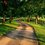# [Analytic Number Theory] Deriving Machin's Formula using Arguments of Complex Numbers

We can derive Machin's formula by inspecting the complex number relation $(5+i)^4 = 2 (1+i) (239 + i)$ (1).

Essentially, what we are doing is relating each quantity of Machin's formula to the argument of a complex number.

First, we recall that the sum of arguments of complex numbers is the argument of their product, and the argument of a complex number raised to a power is simply that number times the argument. That is, the relations $Arg(z,w) = Arg(z) + Arg(w)$, and $Arg(z^k) = k( Arg(z))$ hold for all $z \in \mathbb{C}$.

Taking the argument of both sides of (1), we get $Arg((5+i)^4) = Arg( 2(1+i)(239+i))$ $4(Arg(5+i)) = Arg (2(1+i)) + Arg(239+i)$ $4(Arg(5+i)) -Arg(239+i)= Arg (2(1+i))$ Since $Arg(a+bi) = \arctan (\frac{b}{a})$, RHS $= \arctan(\frac{2}{2}) = \arctan(1) = \frac{\pi}{4}$.

So we have the following relation: $4 \arctan(\frac{1}{5}) - \arctan(\frac{1}{239}) = \frac{\pi}{4}$ as required.

It is also possible to derive other Machin-like formulas via the same method using complex numbers. You can try deriving the following formulas as an exercise:

Euler's $\frac{\pi}{4} = \arctan \frac{1}{2} + \arctan \frac{1}{3}.$ Hermann's $\frac{\pi}{4} =2 \arctan \frac{1}{2} - \arctan \frac{1}{7}.$ Hutton's $\frac{\pi}{4} = 2\arctan \frac{1}{3} + \arctan \frac{1}{7}.$Note by Bright Glow
3 years, 2 months ago

This discussion board is a place to discuss our Daily Challenges and the math and science related to those challenges. Explanations are more than just a solution — they should explain the steps and thinking strategies that you used to obtain the solution. Comments should further the discussion of math and science.

When posting on Brilliant:

• Use the emojis to react to an explanation, whether you're congratulating a job well done , or just really confused .
• Ask specific questions about the challenge or the steps in somebody's explanation. Well-posed questions can add a lot to the discussion, but posting "I don't understand!" doesn't help anyone.
• Try to contribute something new to the discussion, whether it is an extension, generalization or other idea related to the challenge.

MarkdownAppears as
*italics* or _italics_ italics
**bold** or __bold__ bold
- bulleted- list
• bulleted
• list
1. numbered2. list
1. numbered
2. list
Note: you must add a full line of space before and after lists for them to show up correctly
paragraph 1paragraph 2

paragraph 1

paragraph 2

[example link](https://brilliant.org)example link
> This is a quote
This is a quote
    # I indented these lines
# 4 spaces, and now they show
# up as a code block.

print "hello world"
# I indented these lines
# 4 spaces, and now they show
# up as a code block.

print "hello world"
MathAppears as
Remember to wrap math in $$ ... $$ or $ ... $ to ensure proper formatting.
2 \times 3 $2 \times 3$
2^{34} $2^{34}$
a_{i-1} $a_{i-1}$
\frac{2}{3} $\frac{2}{3}$
\sqrt{2} $\sqrt{2}$
\sum_{i=1}^3 $\sum_{i=1}^3$
\sin \theta $\sin \theta$
\boxed{123} $\boxed{123}$